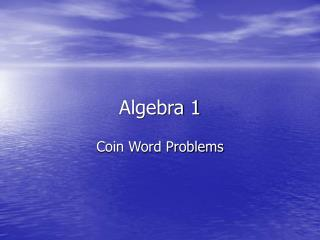DownloadDownload PresentationAlgebra 1

# Algebra 1

Télécharger la présentation## Algebra 1

- - - - - - - - - - - - - - - - - - - - - - - - - - - E N D - - - - - - - - - - - - - - - - - - - - - - - - - - -
##### Presentation Transcript

1. Algebra 1 Coin Word Problems

2. Stan’s pocket is filled with nickels and dimes. He has 6 more dimes than nickels. All together Stan has \$1.20 in coins. How many nickels and dimes does he have? -Let’s start with the sentence “He has 6 more dimes than nickels.” -Ask yourself “Which coin does he have more of?” -The answer is dimes. d = n + 6 Quantity Equation

3. All together Stan has \$1.20 in coins. • Each coin has a different value. • Each nickel is worth 5 cents. Thus 5n is the amount all his nickels are worth. • Each dime is worth 10 cents. Thus 10d is the amount all his dimes are worth. • \$1.20 is worth 120 cents… • 5n + 10d = 120 The value equation.

4. Substitute and solve • 10d + 5n = 120 and d = (n + 6) • 10(n + 6) + 5n = 120 • 10n + 60 + 5n = 120 • 15n + 60 = 120 • 15n = 60 • n = 4

5. Complete the Problem • He has 4 nickels. • d = n + 6 • d = 4 + 6 • d = 10 • Stan has 4 nickels and 6 dimes.

6. Check • 10 dimes is worth 10(.10) = \$1.00 • 4 nickels is worth 4(.05) = \$0.20 Total = \$1.20

7. 4) She gave the sitter 6 more nickels than dimes. • She has more… • Nickels. • n = d + 6 Quantity Equation. • She has 3 times as many quarters as dimes. • She has more… • Quarters. • q =3d Quantity Equation.

8. All together Mrs. Ryan paid the babysitter \$7.50. • Total nickel value in cents… • 5n • Total dime value in cents… • 10d • Total quarter value in cents… • 25q • 5n + 10d + 25q = 750

9. Substitute and Solve • n = d + 6 q = 3d • 5n + 10d + 25q = 750 • 5(d + 6) + 10d + 25(3d) = 750 • 5d + 30 + 10d + 75d = 750 • 90d + 30 = 750 • 90d = 720 • d = 8 • She paid 8 dimes.

10. Solve for Nickels and Quarters • d = 8 • n = d + 6 • n = 8 + 6 • n = 14 • q = 3d • q = 3(8) • q =24

11. Check • 8 dimes is worth 8(\$0.10) = \$0.80 • 14 nickels is worth 14(\$0.05) = \$0.70 • 24 quarters is worth 24(\$0.25) = \$6.00 Total = \$7.50